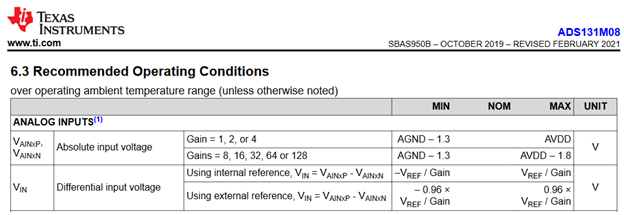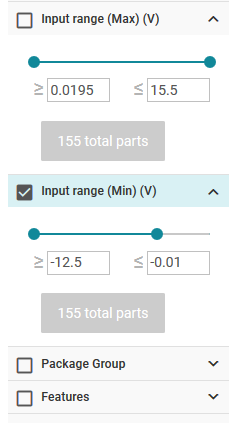If you have a related question, please click the "Ask a related question" button in the top right corner. The newly created question will be automatically linked to this question.

# [FAQ] Can my ADC measure negative voltages?

My ADC’s datasheet gives the input range as ±VREF. Does that mean I can measure negative voltages with respect to ground?

• In order to answer this question, it is first necessary to differentiate between “absolute input voltage” and “differential input voltage”. Both of these specifications may appear in the ADC datasheet, with different considerations for each. Moreover, it is necessary to define what is meant by “ground”, as many ADCs and ADC systems have multiple ground nodes.

Absolute versus differential input voltage

The absolute input voltage (AINx) on an analog input is referenced to a fixed voltage, typically a ground node. As shown in Figure 1, the absolute input voltage for the 24-bit, 8-channel, 30-kSPS ADS1256 is referenced to AGND. While this is common, other ground nodes may include digital ground (DGND) or a negative bipolar supply (AVSS), both of which are discussed later in this document. Refer to the ADC datasheet for specific information regarding the absolute input voltage requirements.

The differential input voltage (VIN) is the measured voltage between two analog inputs. These two inputs are typically denoted positive analog input (AINP) and negative analog input (AINN), such that VIN = AINP – AINN. For single-ended ADCs that only have one input pin, AINN is internally connected to ground such that VIN = AINP. As shown in Figure 1, the differential input voltage range (“full-scale input voltage”) for the ADS1256 is ±2*VREF / PGA. This equation means that the ADC can measure a differential voltage that spans from -2*VREF/gain to +2*VREF/gain.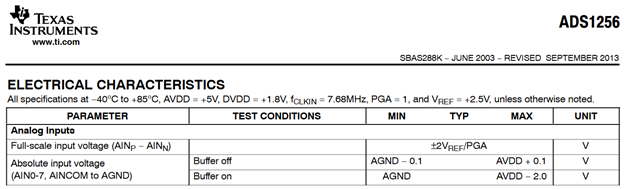As a result, the ADS1256 can measure a negative differential input voltage, though the absolute input voltage on each pin must be positive (≥AGND). For example, if AINP = 5 V and AINN = 0 V, then VIN = AINP – AINN = 5 V – 0 V = +5 V. If AINP and AINN were swapped such that AINP = 0 V and AINN = 5 V, then VIN = 0 V – 5 V = -5 V. This example results in both positive and negative differential input voltages, though – importantly – the absolute input voltage on each pin (AINP and AINN) is always positive. Therefore, the ADS1256 cannot measure negative voltages with respect to ground.

Bipolar power supplies

If measuring negative voltages is required by the system, choosing an ADC with bipolar power supplies could be an option. For example, the Recommended Operating Conditions table for the 24-bit, 12-channel, 4-kSPS ADS124S08 is shown in Figure 2. Specifically, the Analog Power Supply specifications show that AVSS can be negative with respect to digital ground (DGND).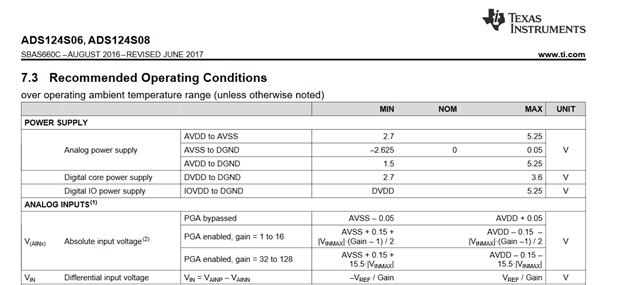A common bipolar supply configuration for the ADS124S08 is AVDD = 2.5 V and AVSS = -2.5 V, both with respect to DGND. When the PGA is bypassed, for example the absolute input range on the ADS124S08 is AVSS – 50 mV < AINx < AVDD + 50 mV. In other words, the ADS124S08 is able to measure negative voltages with respect to DGND if a bipolar analog supply is used. However, if the desired reference point was AVSS – not DGND – then the ADS124S08 cannot measure negative voltages with respect to AVSS, regardless of the supply configuration.

Bipolar input range with a unipolar supply

If the system does not support a bipolar power supply, it is possible to use a bipolar input ADC. For example, the 16-bit, 8-channel, 500-kSPS ADS8688 uses a difference amplifier input structure that allows absolute input voltages of ±10V with respect to ground (AIN_nGND) even when a unipolar power supply is used. Figure 3 shows both the absolute (“operating”) and differential (“full-scale”) input voltage parameters from the ADS8688 datasheet.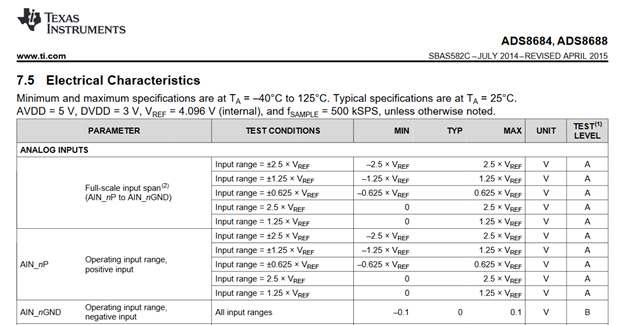Note in Figure 3 that the ADS8688 can measure an absolute input voltage as low as -2.5 * VREF referenced to AIN_nGND. This translates to -10.24 V using the ADC internal 4.096-V voltage reference. Ultimately, the ADS8688 is able to measure negative voltages with respect to ground even when operating on a unipolar supply.

Other ADCs may employ different power structures that can enable measurement of negative voltages. For example, the 24-bit, 8-channel (simultaneous), 32-kSPS ADS131M08 has an integrated charge pump to extend the absolute input range. Figure 4 shows the Analog Input specifications from the ADS131M08 datasheet, where AINP or AINN can be as low as -1.3 V with respect to AGND. While not shown in Figure 4, these specifications assume a unipolar supply.